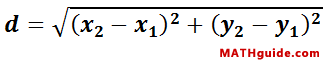Classifying Parallelograms Using Slope and Distance
View the Lesson | MATHguide homepage Updated June 22nd, 2023

Given:Quadrilateral A(-8, 2), B(-2, 4), C(-3, 7), D(-9, 5)Problem:Determine the slope of the diagonals and the length of the diagonals to best classify quadrilateral ABCD.

Slope of Diagonals
 mAC =mBD =Length of Diagonals
 dAC =
 dBD =

Classification: